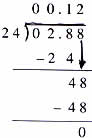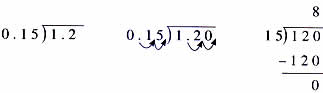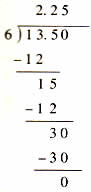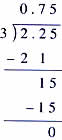Thursday , July 7 2022# NCERT 5th Class (CBSE) Mathematics: Decimals

### Use a shortcut!

Move both decimal points in the dividend and in the divisor by the same number of places till the divisor is a whole number.

(a) 0.288÷2.4 = ?

Step 1.   2.4 ) 0.288 (

Step 3.      24 ) 02.88 ((b)1.2÷o.15## USING MULTIPLICATION AND DIVISION OF DECIMALS

### Unitary Method

(a) Aastha needs to buy some party balloons. A box of 6 balloons is available for Rs. 13.50. A box of 12 balloons is available for Rs. 25.

If both boxes are of the same quality, which box is the batter buy? In other words which of the two boxes has cheaper balloons?

To find out, Aastha needs to find the price of one balloon in a box of 6, and one balloon in a box of 12.

1. To find the price of one balloon in the box of 6.
6 balloons cost Rs. 13.50
What is the cost of 1 balloon? Divide to find out.13.50÷6 = 2.25
One balloon in the box of 6 costs Rs. 2.25
2. To find the price of one balloon in the box of 12.
12 balloons cost Rs. 25.
1 balloon costs 25÷12.
25÷12 = 2.083
2.083 rounded to the nearest hundredths = 2.08
1 balloon in the box of 12 costs Rs. 2.08.

Answer: Since Rs 2.08 is less than Rs 2.25 Aatha buys the packet of 12 balloons because it is the better buy,

(b) Three eraser cost Rs. 2.25. How much will 10 erasers cost?

Step 1. Find out the price of one eraser.

One eraser costs Rs. 0.75Step 2. To find the coast of 10 erasers, multiply the price of one eraser by 10.

0.75×10 = 7.5

10 erasers will cost Rs. 7.50

## चुनौती हिमालय की 5th NCERT CBSE Hindi Rimjhim Ch 18

चुनौती हिमालय की 5th Class NCERT CBSE Hindi Book Rimjhim Chapter 18 प्रश्न: लद्दाख जम्मू-कश्मीर राज्य में …